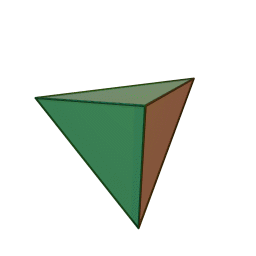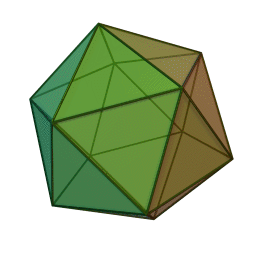# Symmetries of Platonic Solids

Definition: A polyhedron is regular if all its faces are the same regular polygon, and all angles are equal.

It turns out that there are only five Platonic solids (convex regular polyhedra).

Here’s a quick proof:

• For a regular n-gon, the sum of its interior angles is (n – 2)π.
• So the angle at any one of its corners must be (n – 2) π/n.
• Now, suppose we have r faces (each a regular n-gon) meeting at every vertex.
• Since the meeting point for each face is a corner with angle (n – 2)π/n, we have that the sum of the angles is r (n – 2) π/n.
• And since the polyhedron is convex we must have r (n – 2) π/n < 2π.
• This can be rewritten as (r – 2)(n – 2) < 4.
• The only positive integers satisfying this are (nr) = (3, 3), (4, 3), (3, 4), (5, 3), (3, 5).
• These are our five Platonic solids!

(3,3): TetrahedronSymmetry group: S4

(4,3): CubeSymmetry group: S4 × 2

(3,4): OctahedronSymmetry group: S4 × 2

(5,3): DodecahedronSymmetry group: A5 × 2

(3,5): IcosahedronSymmetry group: A5 × 2

— — —

Here’s one more thing to notice; when looking at small dihedral groups, we saw that Dih3 (the symmetry group for a regular triangle) was isomorphic to S3 (the set of permutations of three items). We also saw that this isomorphism did not hold for higher order dihedral groups. In other words, the only regular polygon whose symmetry group was some symmetric group was the triangle.

Now we see that the tetrahedron (which we might consider to be the closest thing to a three-dimensional version of a regular triangle) has as its symmetry group S4. In other words, we get to S4 not by moving to polygons with more sides, but by moving to a higher dimension!

This may bring a conjecture to our minds: perhaps in general, moving up dimensions is the way to get regular geometric shapes that are isomorphic to higher order symmetric groups. That is, perhaps the symmetry group of a regular “triangle-like” objects in n dimensions is isomorphic to Sn. So this would predict that there is some 4D version of the 2D triangle and 3D tetrahedron whose symmetry group is S5. And guess what; this turns out to be right!

The higher dimensional generalization of a triangle and tetrahedron is called a n-simplex. And the symmetry group for each of these simplexes is in fact a symmetric group! So all symmetric groups can be considered to be descriptions of the set of symmetries of some possibly very high-dimensional tetrahedron! This is a fun little piece of trivia that I don’t know quite what to make of.